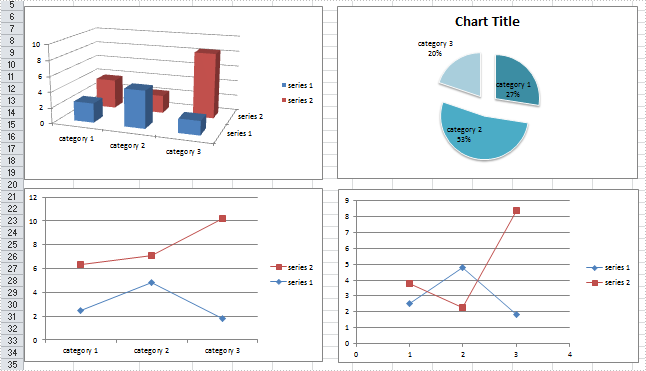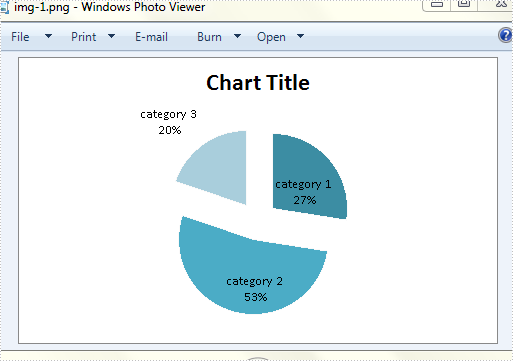# How to Save Excel Charts as Images in C#, VB.NET

An Excel chart is a graphical representation of numbers, which visualizes your data in selected data table. Sometimes, we create a chart with MS Excel, but we don't really want to share the whole Excel file except for the chart. In such a case, we can export charts as image files for easy sharing. In the following section, you will learn how to save your Excel chart as an image in C# and VB.NET via Spire.XLS.

In the test file, I have created four different type of charts based on a same data table. Then, let’s see how each chart can be saved as an image with code.

Test File:#### Code Snippet:

Step 1: Create a new workbook and load the test file.

```Workbook workbook = new Workbook();
```

Step 2: Get the worksheet that contains the chart from workbook.

```Worksheet sheet=workbook.Worksheets;
```

Step 3: Initialize a new instance of image array to store the Bitmap images which are converted from charts.

```Image[] imgs = workbook.SaveChartAsImage(sheet);
```

Step 4: Traverse every item in image array and save them to specified image format.

```for (int i = 0; i < imgs.Length; i++)
{
imgs[i].Save(string.Format("img-{0}.png", i), ImageFormat.Png);
}
```

Output:

Charts have been saved as images in bin folder.The second chart looks like:Full Code:

[C#]
```using Spire.Xls;
using System.Drawing;
using System.Drawing.Imaging;
namespace SaveExcelCharts
{
class Program
{

static void Main(string[] args)
{

Workbook workbook = new Workbook();
Worksheet sheet = workbook.Worksheets;
Image[] imgs = workbook.SaveChartAsImage(sheet);
for (int i = 0; i < imgs.Length; i++)
{
imgs[i].Save(string.Format("img-{0}.png", i), ImageFormat.Png);
}

}
}
}
```
[VB.NET]
```Imports Spire.Xls
Imports System.Drawing
Imports System.Drawing.Imaging
Namespace SaveExcelCharts
Class Program

Private Shared Sub Main(args As String())

Dim workbook As New Workbook()